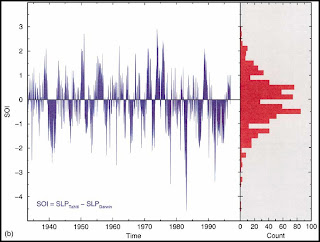# outsidethecube

Name:

## Saturday, May 31, 2008

Climate Science a Brain of two halves.

Our brain has two halves: one is responsible for the multiplication of polynomials and languages, and the other half is responsible for orientation of figures in space and all the things important in real life. Mathematics is geometry when you have to use both halves.

Describing the mathematical problems (some years before the celebrated Hilbert’s list) Poincare divided them into two parts: the binary problems (similar to the Fermat problem, where the answer is a choice between the two possibilities: “yes” or “no”), and the interesting problems, where the progress is continuous, studying first of all the possibility of the variations of the problem (like, say, the variation of the boundary conditions for a differential equation) and investigating then the influences of these variations on the properties of the solutions (which would be hidden, if the problem were formulated as a binary one).

Vladimir Arnold in Bifurcation theory reduces it to an easy understanding.

Poincare bifurcation theory was elaborated by the Russian mathematicians Pontryagin
and Andronov already in the 20’s and in the 30’s (due to the need to apply these bifurcations to radiophysics). Andronov published (with all the proofs) the theory of the birth of a periodic motion of a dynamical system under the generic loss of stability of an equilibrium position, in the case when two eigenvalues of the linearised system cross the imaginary axis, moving from the stable to the unstable complex half-plane.

Andronov’s theorem claims that (depending on the sign of some higher term of the Taylor series) exactly two generic cases may occur: Either the stability of the equilibrium position is inherited by the new-born limit cycle (whose radius grows like the square root of the difference between the new value of the parameter and the value at the stability loss), or else the radius of the attraction domain, diminishing like the square root of the difference between the growing parameter value and the future value, at which the stability will be destroyed, disappears at the stability loss moment.

The first case is called the mild stability loss, the new-born periodic motion-attractor describes a small oscillation near the old stationary regime. The second case is called the hard stability loss, the behaviour of the system after this stability loss being very far from the equilibrium, loosing its stability. The proofs of these results of Andronov on the phase portraits bifurcations were based on the Pontryagin’s extension of Poincare’s results in the holomorphic case to that of the smooth systems of differential equations.As we see with quasiperiodic systems the return to a “previous climate” state is regularly seen in the NAO.,PDO and IPO. These are sates with long periodicity for a climate regime of warmer then normal or cooler then normal climate states.

The ability for a recurrent periodic state such as the PDO or an inverse temperature
“state” is in essence a binary transformation or bifurcation.. The transformation as a velocity inversion has the same effect as a time inversion,(v to -v ) (t to -t)
An interesting property is the probability P+=P- = 0.5 To put this in perspective, for records generated by statistically independent processes with finite standard deviation for periodic bifurcations , where there can be either hard or soft stability loss the exponent is ½.

A fundamental consequence of the aperiodicity of the atmospheric and climate dynamics is the well-known difficulty to make reliable predictions.Contrary to simple periodic or multiperiodic phenomena(such as eclipses,tides ets) for which a long term prediction is possible, predictions in meteorology and climate are limited in time.

The most plausible (and currently admitted) explanation is based on the realization that a small uncertainty in the initial conditions used in a prediction scheme (usually referred as \error") seems to be amplified in the course of the evolution. Such uncertainties are inherent in the process of experimental measurement, The uncertainty being in the “closeness of the boundary to the point of bifurcation, and the error in linear equations.” An important aspect discussed by Arnold and Shuliminov.

As the “natural variability” and inverse regimes are often accompanied by inverse temperature states always and everywhere(except in GCM PREDICTIONS) one wonders if we have a problem of cataclysmic proportions.Erl said...

Max,
All this mathematics gives me a headache. What I see is a period of Arctic warming in the 1940s with as much cooling between 1950 and 1976 as there has been warming since that date. It is important to realize that the temperature change is occurring in winter.

Now, what determines winter temperature is the residual heat in the ocean after summer. Only equator-wards of 40° Lat. does energy gain from insolation exceed loss via radiation.

The Arctic can be warmed from the atmosphere or the ocean.

There is not much warmth to be had from the atmosphere.

It follows as a matter of logic that the flux in energy gained in the tropics is the phenomenon behind these oceanic oscillations that drive winter temperature at high latitudes.

8:12 PMWeb Counters